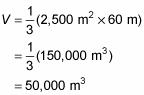##### Basic Math & Pre-Algebra All-in-One For Dummies (+ Chapter Quizzes Online)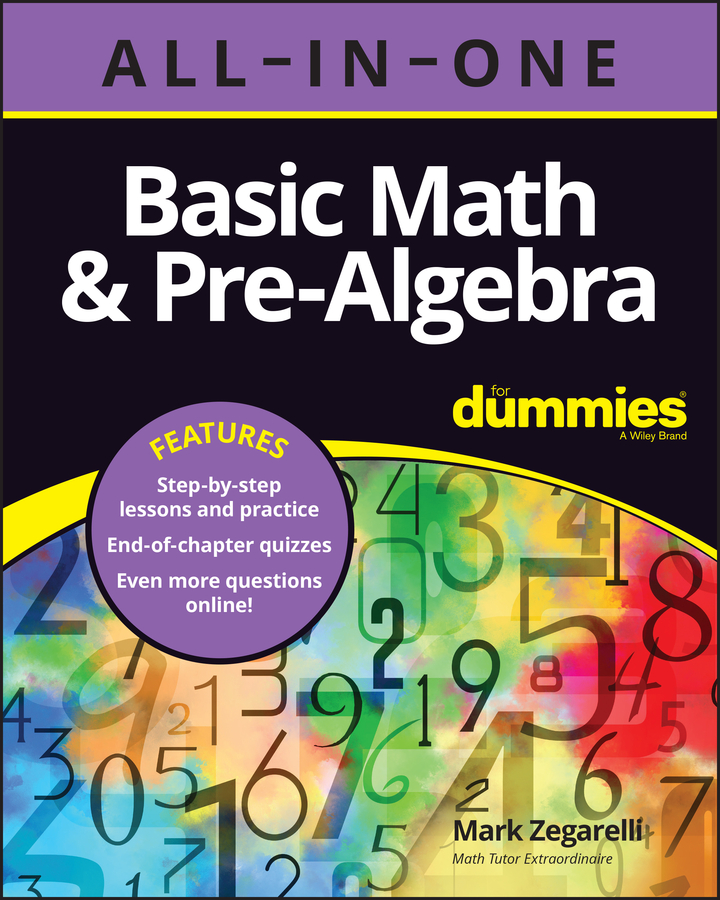Measuring in three dimensions is similar to measuring in two dimensions; however, in 3-D, the boundary of a solid is called its surface area (not its perimeter) and what’s inside a solid is called its volume (not its area).

The surface area of a solid is a measurement of the size of its surface, as measured in square units such as square inches (in.2), square feet (ft.2), square meters (m2), and so forth. The volume (V) of a solid is a measurement of the space it occupies, as measured in cubic units such as cubic inches (in.3), cubic feet (ft.3), cubic meters (m3), and so forth.

## Measuring spheres

The center of a sphere is a point that’s the same distance from any point on the sphere itself. This distance is called the radius (r) of the sphere. If you know the radius of a sphere, you can find out its volume using the following formula: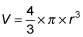Because this formula includes p, using 3.14 as an approximate value for p gives you an approximation of the volume. For example, here’s how to figure out the approximate volume of a ball whose radius is 4 inches:(Note: In the preceding problem, you use equal signs when a value is equal to whatever comes right before it and approximately-equal-to signs () when you round.)

## Measuring cubes

The main measurement of a cube is the length of its side (s). Using this measurement, you can find out the volume of a cube using the following formula:

V = s3

So if the side of a cube is 5 meters, here’s how you figure out its volume:

V = (5 m)3 = 5 m 5 m 5 m = 125 m3

You can read 125 m3 as 125 cubic meters or, less commonly, as 125 meters cubed.

## Measuring boxes (rectangular solids)

The three measurements of a box (or rectangular solid) are its length (l), width (w), and height (h). The box pictured in the figure below has the following measurements:

l = 4 m, w = 3 m, and h = 2 m.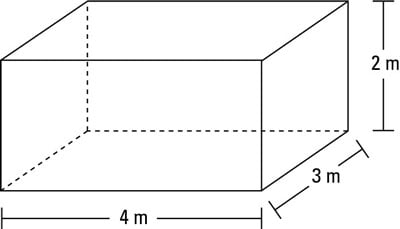You can find the volume of a box using the following formula:

V = l w h

So here’s how to find the volume of the box pictured above:

V = 4 m 3 m 2 m = 24 m3

## Measuring prisms

Finding the volume of a prism is easy if you have two measurements. One measurement is the height (h) of the prism. The second is the area of the base (Ab). The base is the polygon that extends vertically from the plane.

Here’s the formula for finding the volume of a prism:

V = Ab h

For example, suppose a prism has a base with an area of 5 square centimeters and a height of 3 centimeters. Here’s how you find its volume:

V = 5 cm2 3 cm = 15 cm3

Notice that the units of measurements (cm2 and cm) are also multiplied, giving you a result of cm3.

## Measuring cylinders

You find the volume of cylinders the same way you find the area of prisms — by multiplying the area of the base (Ab) by the cylinder’s height (h):

V = Ab h

Suppose you want to find the volume of a cylindrical can whose height is 4 inches and whose base is a circle with a radius of 2 inches. First, find the area of the base by using the formula for the area of a circle:

Ab = p r2

3.14 (2 in.)2

= 3.14 4 in.2

= 12.56 in.2

This area is approximate because you use 3.14 as an approximate value for p.

Now use this area to find the volume of the cylinder:

V 12.56 in.2 4 in. = 50.24 in.3

Notice how multiplying square inches (in.2) by inches gives a result in cubic inches (in.3).

## Measuring pyramids and cones

The two key measurements for pyramids and cones are the same as those for prisms and cylinders: the height (h) and the area of the base (Ab). Here’s the formula for the volume of a pyramid or a cone: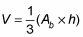For example, suppose you want to find the volume of an ice cream cone whose height is 4 inches and whose base area is 3 square inches. Here’s how you do it: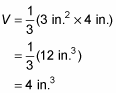Similarly, suppose you want to find the volume of a pyramid in Egypt whose height is 60 meters with a square base whose sides are each 50 meters. First, find the area of the base:

Ab = s2 = (50 m)2 = 2,500 m2

Now use this area to find the volume of the pyramid: